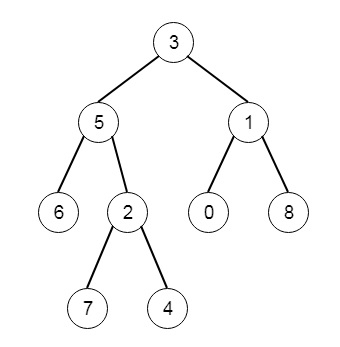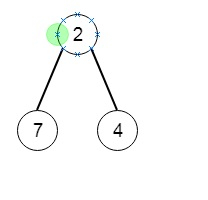# Smallest Subtree with all the Deepest Nodes in C++

Suppose we have a binary tree rooted at root, the depth of each node is the shortest distance to the root. Here a node is deepest if it has the largest depth possible among any node in the entire tree. The subtree of a node is that node, plus the set of all descendants of that node. We have to find the node with the largest depth such that it contains all the deepest nodes in its subtree. So if the tree is like −Then the deepest subtree will be −To solve this, we will follow these steps −

• Define a method called solve(), this will take root as input

• if root is null, then return (null, 0)

• l := solve(left of root), r := solve(right of root)

• if second value of left > second value of r, then return a pair (first of l, 1 + second of l)

• otherwise when second value of left < second value of r, then return a pair (first of r and 1 + second of r)

• return a pair(root, second of l + 1)

• From the main method call solve(root), and return the second value of it

## Example(C++)

Let us see the following implementation to get a better understanding −

Live Demo

#include <bits/stdc++.h>
using namespace std;
class TreeNode{
public:
int val;
TreeNode *left, *right;
TreeNode(int data){
val = data;
left = right = NULL;
}
};
void insert(TreeNode **root, int val){
queue<TreeNode*> q;
q.push(*root);
while(q.size()){
TreeNode *temp = q.front();
q.pop();
if(!temp->left){
if(val != NULL)
temp->left = new TreeNode(val);
else
temp->left = new TreeNode(0);
return;
} else {
q.push(temp->left);
}
if(!temp->right){
if(val != NULL)
temp->right = new TreeNode(val);
else
temp->right = new TreeNode(0);
return;
} else {
q.push(temp->right);
}
}
}
TreeNode *make_tree(vector<int> v){
TreeNode *root = new TreeNode(v);
for(int i = 1; i<v.size(); i++){
insert(&root, v[i]);
}
return root;
}
void tree_level_trav(TreeNode*root){
if (root == NULL) return;
cout << "[";
queue<TreeNode *> q;
TreeNode *curr;
q.push(root);
q.push(NULL);
while (q.size() > 1) {
curr = q.front();
q.pop();
if (curr == NULL){
q.push(NULL);
} else {
if(curr->left)
q.push(curr->left);
if(curr->right)
q.push(curr->right);
if(curr->val == 0 || curr == NULL){
cout << "null" << ", ";
} else {
cout << curr->val << ", ";
}
}
}
cout << "]"<<endl;
}
class Solution {
public:
pair <TreeNode*, int> solve(TreeNode* root){
if(!root || root->val == 0) return {NULL, 0};
pair <TreeNode*, int> L = solve(root->left);
pair <TreeNode*, int> R = solve(root->right);
if(L.second > R.second)return {L.first, L.second + 1};
else if(L.second < R.second) return {R.first, R.second + 1};
return {root, L.second + 1};
}
TreeNode* subtreeWithAllDeepest(TreeNode* root) {
return solve(root).first;
}
};
main(){
vector<int> v = {3,5,1,6,2,0,8,NULL,NULL,7,4};
TreeNode *root = make_tree(v);
Solution ob;
tree_level_trav(ob.subtreeWithAllDeepest(root)) ;
}

## Input

{3,5,1,6,2,0,8,NULL,NULL,7,4}

## Output

[2,7,4]

Updated on: 30-Apr-2020

58 Views